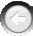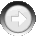﻿ Quick Function Reference Chart

# Quick Function Reference Chart

Navigation:  »No topics above this level«

# Quick Function Reference ChartCalcMeanFitness(Population As Integer, MeanFitness As Single) CalcOrderArrayPath(NumOfPoints As Integer, OrderArray As Integer, Xarray As Single,Yarray As Single, Path As Single) FindBest(Population As Integer, BestIndividual As Integer, BestFitness As Single) GetChromosome(Population As Integer, Individual As Integer, Chromosome As Integer, ChromoValue As Single) GetChromosomePool(Population As Integer, Individual As Integer, ChromoPool As Integer, PoolArray As Single) GetEnumChromosome(Population As Integer, Individual As Integer, EnumChromosome As Integer, ChromosomeArray As Integer) GetEnumChromosomeParm(Population As Integer, EnumChromosome As Integer, EnumChromoRange As Integer, EnumChromoLength As Integer) GetFitness(Population As Integer, Individual As Integer, Fitness As Single) GetMaxPopulNum(MaxPopulationNum As Integer) GetNextPopulation(Population As Integer) GetPopulParam(Population As Integer, GenerationNum As Integer, PopulationSize As Integer, TotalChromo As Integer, TotalPool As Integer, TotalEnumChromo As Integer) KillPopulation(Population As Integer) MakeChromosome(Population As Integer, Chromosome As Integer, Precision As Integer, MaxValue As Single, MinValue As Single, IntType as Integer) MakeChromosomePool(Population As Integer, ChromoPool As Integer, PoolSize As Integer, Precision As Integer, MaxValue As Single, MinValue As Single) MakeEnumChromosome(Population As Integer, EnumChromosome As Integer, Range As Integer, Length As Integer, Type As Integer) MakePopulation(Population As Integer, PopSize As Integer, SerialNo As Long) PutChromosome(Population As Integer, Individual As Integer, Chromosome As Integer, ChromoValue As Single) PutChromosomePool(Population As Integer, Individual As Integer, ChromoPool As Integer, PoolArray As Single) PutEnumChromosome(Population As Integer, Individual As Integer, EnumChromosome As Integer, ChromosomeArray As Integer) PutFitness(Population As Integer, Individual As Integer, Fitness As Single) Reproduce(Population As Integer) SetDiversity(Population as Integer, DiversityRate as Single, DiversityRadius as Single) SetEnumOperators(Population As Integer, CrossoverType As Integer, EnumCrossoverRate As Single, EnumMutationRate As Single) SetExtinction(Population as Integer, ExtinctionPeriod as Integer, ExtinctionRate as Single) SetGenSeed(Seed As Integer) SetOperators(Population As Integer, CrossoverType As Integer, CrossoverRate As Single, MutationRate As Single) SetStrategy(Population As Integer, SelectionType As Integer, GenerationGap As Single, FitnessRatio As Single)